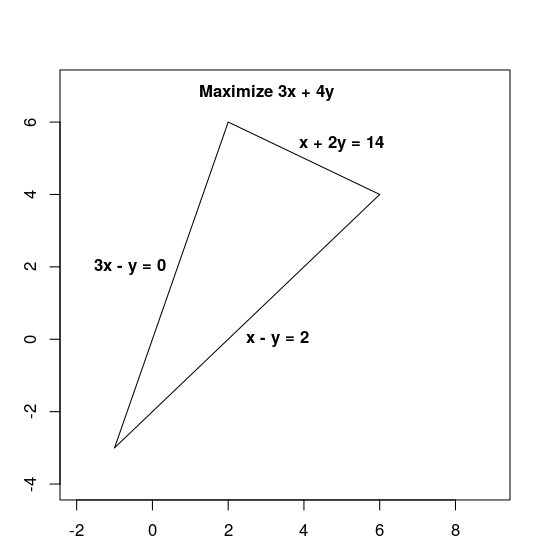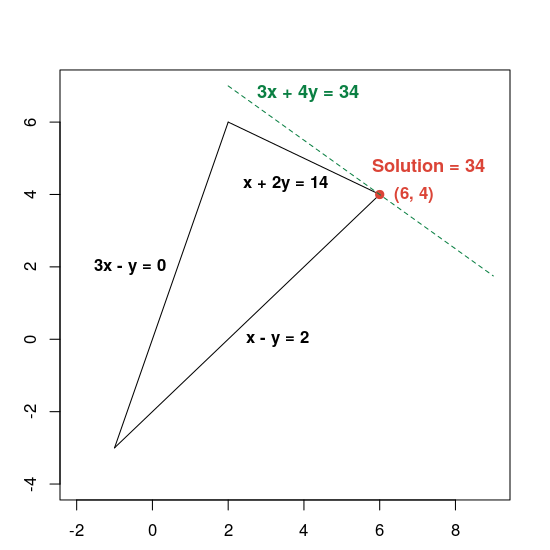# Solving an LP Problem

The following sections present an example of an LP problem and show how to solve it. Here's the problem:

Maximize 3x + 4y subject to the following constraints:
 x + 2y ≤ 14 3x – y ≥ 0 x – y ≤ 2

Both the objective function, 3x + 4y, and the constraints are given by linear expressions, which makes this a linear problem.

The constraints define the feasible region, which is the triangle shown below, including its interior.## Basic steps for solving an LP problem

To solve a LP problem, your program should include the following steps :

1. Import the linear solver wrapper,
2. declare the LP solver,
3. define the variables,
4. define the constraints,
5. define the objective,
6. call the LP solver; and
7. display the solution

## Solution using the MPSolver

The following section present a program that solves the problem using the MPSolver wrapper and an LP solver.

Note. To run the program below, you need to install OR-Tools.

The primary OR-Tools linear optimization solver is Glop, Google's in-house linear programming solver. It's fast, memory efficient, and numerically stable.

### Import the linear solver wrapper

Import (or include) the OR-Tools linear solver wrapper, an interface for MIP solvers and linear solvers, as shown below.

### Python

from ortools.linear_solver import pywraplp

### C++

#include <iostream>

#include "ortools/linear_solver/linear_solver.h"

### Java

import com.google.ortools.Loader;
import com.google.ortools.linearsolver.MPVariable;

### C#

using System;
using Google.OrTools.LinearSolver;

### Declare the LP solver

MPsolver is a wrapper for several different solvers, including Glop. The code below declares the GLOP solver.

### Python

solver = pywraplp.Solver.CreateSolver('GLOP')

### C++

std::unique_ptr<MPSolver> solver(MPSolver::CreateSolver("SCIP"));
if (!solver) {
LOG(WARNING) << "SCIP solver unavailable.";
return;
}

### Java

MPSolver solver = MPSolver.createSolver("GLOP");

### C#

Solver solver = Solver.CreateSolver("GLOP");

Note: If you install OR-Tools from source, there are other third-party solvers available. See the "Install from Source" section of the installation guide for your platform.

### Create the variables

First, create variables x and y whose values are in the range from 0 to infinity.

### Python

x = solver.NumVar(0, solver.infinity(), 'x')
y = solver.NumVar(0, solver.infinity(), 'y')

print('Number of variables =', solver.NumVariables())

### C++

const double infinity = solver->infinity();
// x and y are non-negative variables.
MPVariable* const x = solver->MakeNumVar(0.0, infinity, "x");
MPVariable* const y = solver->MakeNumVar(0.0, infinity, "y");
LOG(INFO) << "Number of variables = " << solver->NumVariables();

### Java

double infinity = java.lang.Double.POSITIVE_INFINITY;
// x and y are continuous non-negative variables.
MPVariable x = solver.makeNumVar(0.0, infinity, "x");
MPVariable y = solver.makeNumVar(0.0, infinity, "y");
System.out.println("Number of variables = " + solver.numVariables());

### C#

Variable x = solver.MakeNumVar(0.0, double.PositiveInfinity, "x");
Variable y = solver.MakeNumVar(0.0, double.PositiveInfinity, "y");

Console.WriteLine("Number of variables = " + solver.NumVariables());

### Define the constraints

Next, define the constraints on the variables. Give each constraint a unique name (such as constraint0), and then define the coefficients for the constraint.

### Python

# Constraint 0: x + 2y <= 14.
solver.Add(x + 2 * y <= 14.0)

# Constraint 1: 3x - y >= 0.
solver.Add(3 * x - y >= 0.0)

# Constraint 2: x - y <= 2.

print('Number of constraints =', solver.NumConstraints())

### C++

// x + 2*y <= 14.
MPConstraint* const c0 = solver->MakeRowConstraint(-infinity, 14.0);
c0->SetCoefficient(x, 1);
c0->SetCoefficient(y, 2);

// 3*x - y >= 0.
MPConstraint* const c1 = solver->MakeRowConstraint(0.0, infinity);
c1->SetCoefficient(x, 3);
c1->SetCoefficient(y, -1);

// x - y <= 2.
MPConstraint* const c2 = solver->MakeRowConstraint(-infinity, 2.0);
c2->SetCoefficient(x, 1);
c2->SetCoefficient(y, -1);
LOG(INFO) << "Number of constraints = " << solver->NumConstraints();

### Java

// x + 2*y <= 14.
MPConstraint c0 = solver.makeConstraint(-infinity, 14.0, "c0");
c0.setCoefficient(x, 1);
c0.setCoefficient(y, 2);

// 3*x - y >= 0.
MPConstraint c1 = solver.makeConstraint(0.0, infinity, "c1");
c1.setCoefficient(x, 3);
c1.setCoefficient(y, -1);

// x - y <= 2.
MPConstraint c2 = solver.makeConstraint(-infinity, 2.0, "c2");
c2.setCoefficient(x, 1);
c2.setCoefficient(y, -1);
System.out.println("Number of constraints = " + solver.numConstraints());

### C#

// x + 2y <= 14.
solver.Add(x + 2 * y <= 14.0);

// 3x - y >= 0.
solver.Add(3 * x - y >= 0.0);

// x - y <= 2.

Console.WriteLine("Number of constraints = " + solver.NumConstraints());

### Define the objective function

The following code defines the objective function, 3x + 4y, and specifies that this is a maximization problem.

### Python

# Objective function: 3x + 4y.
solver.Maximize(3 * x + 4 * y)

### C++

// Objective function: 3x + 4y.
MPObjective* const objective = solver->MutableObjective();
objective->SetCoefficient(x, 3);
objective->SetCoefficient(y, 4);
objective->SetMaximization();

### Java

// Maximize 3 * x + 4 * y.
MPObjective objective = solver.objective();
objective.setCoefficient(x, 3);
objective.setCoefficient(y, 4);
objective.setMaximization();

### C#

// Objective function: 3x + 4y.
solver.Maximize(3 * x + 4 * y);

### Invoke the solver

The following code invokes the solver.

### Python

status = solver.Solve()

### C++

const MPSolver::ResultStatus result_status = solver->Solve();
// Check that the problem has an optimal solution.
if (result_status != MPSolver::OPTIMAL) {
LOG(FATAL) << "The problem does not have an optimal solution!";
}

### Java

final MPSolver.ResultStatus resultStatus = solver.solve();

### C#

Solver.ResultStatus resultStatus = solver.Solve();

### Display the solution

The following code displays the solution.

### Python

if status == pywraplp.Solver.OPTIMAL:
print('Solution:')
print('Objective value =', solver.Objective().Value())
print('x =', x.solution_value())
print('y =', y.solution_value())
else:
print('The problem does not have an optimal solution.')

### C++

LOG(INFO) << "Solution:";
LOG(INFO) << "Optimal objective value = " << objective->Value();
LOG(INFO) << x->name() << " = " << x->solution_value();
LOG(INFO) << y->name() << " = " << y->solution_value();

### Java

if (resultStatus == MPSolver.ResultStatus.OPTIMAL) {
System.out.println("Solution:");
System.out.println("Objective value = " + objective.value());
System.out.println("x = " + x.solutionValue());
System.out.println("y = " + y.solutionValue());
} else {
System.err.println("The problem does not have an optimal solution!");
}

### C#

// Check that the problem has an optimal solution.
if (resultStatus != Solver.ResultStatus.OPTIMAL)
{
Console.WriteLine("The problem does not have an optimal solution!");
return;
}
Console.WriteLine("Solution:");
Console.WriteLine("Objective value = " + solver.Objective().Value());
Console.WriteLine("x = " + x.SolutionValue());
Console.WriteLine("y = " + y.SolutionValue());

### The complete programs

The complete programs are shown below.

### Python

from ortools.linear_solver import pywraplp

def LinearProgrammingExample():
"""Linear programming sample."""
# Instantiate a Glop solver, naming it LinearExample.
solver = pywraplp.Solver.CreateSolver('GLOP')

# Create the two variables and let them take on any non-negative value.
x = solver.NumVar(0, solver.infinity(), 'x')
y = solver.NumVar(0, solver.infinity(), 'y')

print('Number of variables =', solver.NumVariables())

# Constraint 0: x + 2y <= 14.
solver.Add(x + 2 * y <= 14.0)

# Constraint 1: 3x - y >= 0.
solver.Add(3 * x - y >= 0.0)

# Constraint 2: x - y <= 2.

print('Number of constraints =', solver.NumConstraints())

# Objective function: 3x + 4y.
solver.Maximize(3 * x + 4 * y)

# Solve the system.
status = solver.Solve()

if status == pywraplp.Solver.OPTIMAL:
print('Solution:')
print('Objective value =', solver.Objective().Value())
print('x =', x.solution_value())
print('y =', y.solution_value())
else:
print('The problem does not have an optimal solution.')

print('Problem solved in %f milliseconds' % solver.wall_time())
print('Problem solved in %d iterations' % solver.iterations())

LinearProgrammingExample()

### C++

#include <iostream>

#include "ortools/linear_solver/linear_solver.h"

namespace operations_research {
void LinearProgrammingExample() {
std::unique_ptr<MPSolver> solver(MPSolver::CreateSolver("SCIP"));
if (!solver) {
LOG(WARNING) << "SCIP solver unavailable.";
return;
}

const double infinity = solver->infinity();
// x and y are non-negative variables.
MPVariable* const x = solver->MakeNumVar(0.0, infinity, "x");
MPVariable* const y = solver->MakeNumVar(0.0, infinity, "y");
LOG(INFO) << "Number of variables = " << solver->NumVariables();

// x + 2*y <= 14.
MPConstraint* const c0 = solver->MakeRowConstraint(-infinity, 14.0);
c0->SetCoefficient(x, 1);
c0->SetCoefficient(y, 2);

// 3*x - y >= 0.
MPConstraint* const c1 = solver->MakeRowConstraint(0.0, infinity);
c1->SetCoefficient(x, 3);
c1->SetCoefficient(y, -1);

// x - y <= 2.
MPConstraint* const c2 = solver->MakeRowConstraint(-infinity, 2.0);
c2->SetCoefficient(x, 1);
c2->SetCoefficient(y, -1);
LOG(INFO) << "Number of constraints = " << solver->NumConstraints();

// Objective function: 3x + 4y.
MPObjective* const objective = solver->MutableObjective();
objective->SetCoefficient(x, 3);
objective->SetCoefficient(y, 4);
objective->SetMaximization();

const MPSolver::ResultStatus result_status = solver->Solve();
// Check that the problem has an optimal solution.
if (result_status != MPSolver::OPTIMAL) {
LOG(FATAL) << "The problem does not have an optimal solution!";
}

LOG(INFO) << "Solution:";
LOG(INFO) << "Optimal objective value = " << objective->Value();
LOG(INFO) << x->name() << " = " << x->solution_value();
LOG(INFO) << y->name() << " = " << y->solution_value();
}
}  // namespace operations_research

int main(int argc, char** argv) {
operations_research::LinearProgrammingExample();
return EXIT_SUCCESS;
}

### Java

package com.google.ortools.linearsolver.samples;

/** Simple linear programming example. */
public final class LinearProgrammingExample {
public static void main(String[] args) {
MPSolver solver = MPSolver.createSolver("GLOP");

double infinity = java.lang.Double.POSITIVE_INFINITY;
// x and y are continuous non-negative variables.
MPVariable x = solver.makeNumVar(0.0, infinity, "x");
MPVariable y = solver.makeNumVar(0.0, infinity, "y");
System.out.println("Number of variables = " + solver.numVariables());

// x + 2*y <= 14.
MPConstraint c0 = solver.makeConstraint(-infinity, 14.0, "c0");
c0.setCoefficient(x, 1);
c0.setCoefficient(y, 2);

// 3*x - y >= 0.
MPConstraint c1 = solver.makeConstraint(0.0, infinity, "c1");
c1.setCoefficient(x, 3);
c1.setCoefficient(y, -1);

// x - y <= 2.
MPConstraint c2 = solver.makeConstraint(-infinity, 2.0, "c2");
c2.setCoefficient(x, 1);
c2.setCoefficient(y, -1);
System.out.println("Number of constraints = " + solver.numConstraints());

// Maximize 3 * x + 4 * y.
MPObjective objective = solver.objective();
objective.setCoefficient(x, 3);
objective.setCoefficient(y, 4);
objective.setMaximization();

final MPSolver.ResultStatus resultStatus = solver.solve();

if (resultStatus == MPSolver.ResultStatus.OPTIMAL) {
System.out.println("Solution:");
System.out.println("Objective value = " + objective.value());
System.out.println("x = " + x.solutionValue());
System.out.println("y = " + y.solutionValue());
} else {
System.err.println("The problem does not have an optimal solution!");
}

System.out.println("Problem solved in " + solver.wallTime() + " milliseconds");
System.out.println("Problem solved in " + solver.iterations() + " iterations");
}

private LinearProgrammingExample() {}
}

### C#

using System;

public class LinearProgrammingExample
{
static void Main()
{
Solver solver = Solver.CreateSolver("GLOP");
// x and y are continuous non-negative variables.
Variable x = solver.MakeNumVar(0.0, double.PositiveInfinity, "x");
Variable y = solver.MakeNumVar(0.0, double.PositiveInfinity, "y");

Console.WriteLine("Number of variables = " + solver.NumVariables());

// x + 2y <= 14.
solver.Add(x + 2 * y <= 14.0);

// 3x - y >= 0.
solver.Add(3 * x - y >= 0.0);

// x - y <= 2.

Console.WriteLine("Number of constraints = " + solver.NumConstraints());

// Objective function: 3x + 4y.
solver.Maximize(3 * x + 4 * y);

Solver.ResultStatus resultStatus = solver.Solve();

// Check that the problem has an optimal solution.
if (resultStatus != Solver.ResultStatus.OPTIMAL)
{
Console.WriteLine("The problem does not have an optimal solution!");
return;
}
Console.WriteLine("Solution:");
Console.WriteLine("Objective value = " + solver.Objective().Value());
Console.WriteLine("x = " + x.SolutionValue());
Console.WriteLine("y = " + y.SolutionValue());

Console.WriteLine("Problem solved in " + solver.WallTime() + " milliseconds");
Console.WriteLine("Problem solved in " + solver.Iterations() + " iterations");
}
}

### Optimal solution

The program returns the optimal solution to the problem, as shown below.

Number of variables = 2
Number of constraints = 3
Solution:
x = 6.0
y = 4.0
Optimal objective value = 34.0

Here is a graph showing the solution:The dashed green line is defined by setting the objective function equal to its optimal value of 34. Any line whose equation has the form 3x + 4y = c is parallel to the dashed line, and 34 is the largest value of c for which the line intersects the feasible region.

If you think about the geometry in the above graph, in any linear optimization problem at least one vertex of the feasible region must be an optimal solution. As a result, you can find an optimal solution by traversing the vertices of the feasible region until there is no more improvement in the objective function. This is the idea behind simplex algorithm, the most widely-used method for solving linear optimization problems.